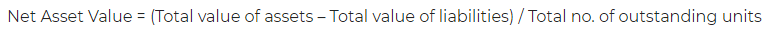# Net Asset Value – Definition, Formula and Example

Net asset value (NAV) refers to the market value of single unit and is calculated by dividing the net assets by total number of outstanding units.

Here,

Net assets = Total assets – Total liabilities

Outstanding units = Units or shares currently held by shareholders or investors

#### Net Asset Value Formula:

The following formula can be used in the computation of NAV:NAV is associated with mutual funds and in that context, it represents market value of single unit of a particular fund. The market value of unit is the price at which unit can be trade in the market.

#### Net Asset Value Example:

Lets assume that Vikas Yadav wants to invest in mutual fund of XYZ Ltd and has the following figures:

Total assets of mutual fund = INR 1,00,000

Total liabilities of mutual fund = INR 30,000

Total outstanding units : 5,000

He can calculate the market value or NAV as below:

Market value or NAV = (1,00,000 – 30,000)/ 5,000 = INR 14

Posted on Categories Dictionary

## One thought on “Net Asset Value – Definition, Formula and Example”

1.Forever says:

So awesome.